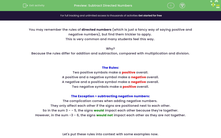# Subtract Directed Numbers

In this worksheet, students will apply the rules related to positive and negative numbers to work out answers to subtraction sums.Key stage:  KS 4

Year:  GCSE

GCSE Subjects:   Maths

GCSE Boards:   Pearson Edexcel, OCR, Eduqas, AQA,

Curriculum topic:   Number, Number Operations and Integers

Curriculum subtopic:   Structure and Calculation Calculations with Integers

Difficulty level:#### Worksheet Overview

You may remember the rules of directed numbers (which is just a fancy way of saying positive and negative numbers), but find them trickier to apply.

This is very common and many students feel this way.

Why?

Because the rules differ for addition and subtraction, compared with multiplication and division.

The Rules:

Two positive symbols make a positive overall.

A positive and a negative symbol make a negative overall.

A negative and a positive symbol make a negative overall.

Two negative symbols make a positive overall.​

The Exception > subtracting negative numbers:

The complication comes when adding negative numbers.

They only affect each other if the signs are positioned next to each other.

So in the sum 3 - - 5, the signs would impact each other because they're together.

However, in the sum -3 - 6, the signs would not impact each other as they are not together.

Let's put these rules into context with some examples now.

e.g. Work out the value of: 6 - + 2

In this question we have a + and a - together, so they will affect each other.

They will become a negative overall.

So we can rewrite the sum as: 6 - 2

If we picture a mental number line, to take 2 from 6, we move 2 spaces to the left on the number line to arrive at: 4

e.g. Work out the value of: - 3 - - 5

In this question we have a - and a - together, so they will affect each other and become a positive overall.

However, the - at the start of the sum is on its own so does not need to be changed at all.

This means we can rewrite the sum as: - 3 + 5

Start at -3 on your mental number line and move 5 spaces to the right (as this is an addition sum) to reach: 2

In this activity, you will apply the rules related to positive and negative numbers to work out answers to subtraction sums.

### What is EdPlace?

We're your National Curriculum aligned online education content provider helping each child succeed in English, maths and science from year 1 to GCSE. With an EdPlace account you’ll be able to track and measure progress, helping each child achieve their best. We build confidence and attainment by personalising each child’s learning at a level that suits them.

Get started